# Exploring Shakti E-Class — HelloWorld, Blinky’s, Donuts and Fixed Point Magic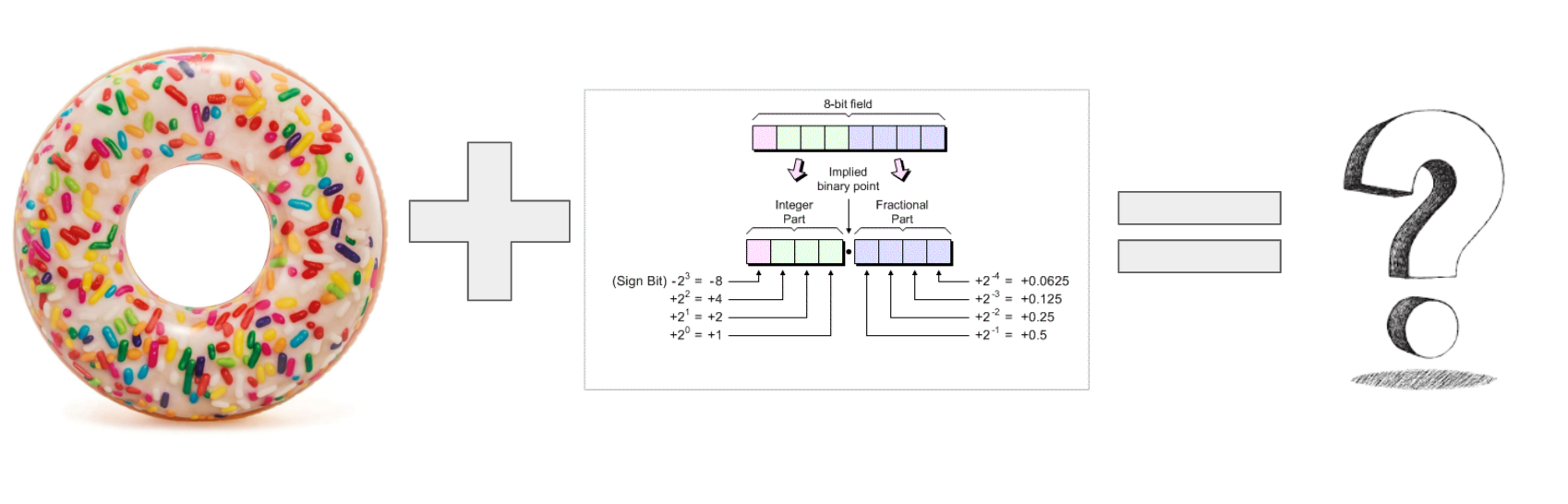No, this really does make sense once you read further...

I apologize for the nearly 4 months long hiatus but outside of general work stuff, I ran into issues with the openocd and shakthi which slowed progress until that got resolved. See here for more details if interested.

Warning: If you have are following instructions from Part-1 where we built the processor, DONOT use the bit file I’ve uploaded in the pre-built repo here; this has been updated in the shakthi repo here; grab that and program it and you should be good to go.

# PlatformIO and Shakti Platform Installation

I’ve decided to use PlatformIO with VSCode for evaluating the Shakti to start with although I will give Zephyr a shot when I can.

There’s an excellent existing guide here already, so I won’t be redoing the instructions here. Pay particular attention to the Drivers/Zadig step, I ran into it and spent many painful hours on it.

# Code repository

All code I made for the below examples is available here, feel free to reuse as you see fit

https://github.com/sreeharshaangara/shakti-eclass-pio-examples

# Hello World Project

From the main page, just click on Project examples, then the uart-hello example and it will pull all the necessary files.

We can build and…. crap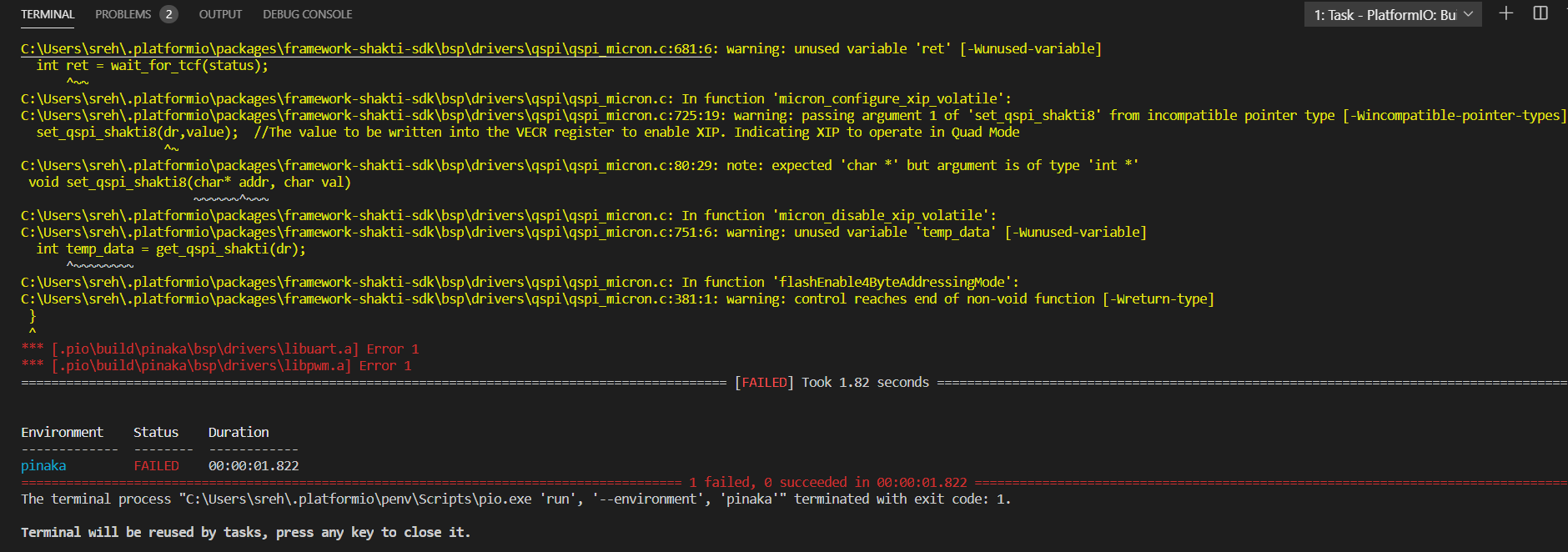Warning hell…

I originally assumed that it was some build variable which I was missing but once I started looking at the code it turns out the drivers really are messed up right now.

In addition, it seems like there is a particular build order issue with the libxadc.a which goes away when you try to rebuild(or clean and rebuild). I’m sure this will get fixed eventually but it isn’t too painful.

And on upload… viola!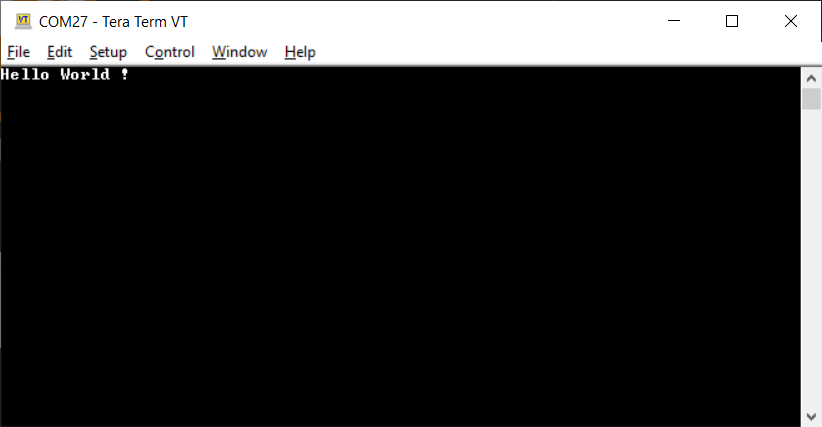Hello world

# Blinking a few LED’s

Now, that that’s done with I wanted to try blinking a few LED’s. There’s an example project using a gpio keypad, but I just wanted to grab a few code snippets and add them.

Unfortunately, the GPIO driver is pretty basic… for most practical purposes I’d say it doesn’t exist. All it had was a definition for the GPIO Data/direction register and little else. Call me spoilt, but I was hoping for atleast write/read API access with the bitmask… sigh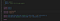I died a little on the inside when I saw this

Much later when I was looking through other code, it turns out there is a led_driver.c/.h available which seemed reasonable. I’d recommend starting there

The User guide here specifies a map of how GPIO’s are were mapped to LED’s and switches.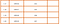GPIO <-> LED Mapping… but this is wrong as we will find out later

And before long viola…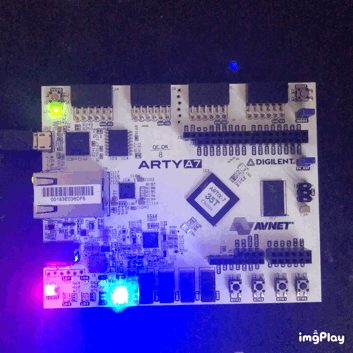Ah.. finally feels like a real embedded project now

# Upgrading the Blinky experience

Well, you know I’ve got 4 LED’s in there so why not make a simple 4-bit up counter.

As I was implementing this, I realized there’s a mistake in the the user guide mappings are not quite right. LED’s 0~4 are actually RGB LED’s with the mapping,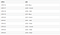The real GPIO LED Mapping

Once I figured that out, it was easy enough to do a LED counter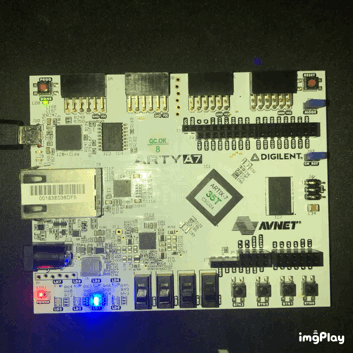A simple 4-bit counter

# Donuts, donuts everywhere

For the final little challenge, I wanted to try out if I could render a toroid(donut) using math functions on the chip.

The original code and idea is from https://www.a1k0n.net/2011/07/20/donut-math.html and is a fascinating read.

Yes, yes I know the E-class is running at a measly 50MHz and doesn’t have a float point accelerator which means it’s pretty much guaranteed to be exceptionally slow without some real optimizations. But hey, where’s the fun if life was that easy eh?

Original source code for printing the donut function is shown below; yes this also prints donuts :)

`k;double sin()         ,cos();main(){float A=       0,B=0,i,j,z;char b[     1760];printf("\x1b[2J");for(;;  ){memset(b,32,1760);memset(z,0,7040)  ;for(j=0;6.28>j;j+=0.07)for(i=0;6.28 >i;i+=0.02){float c=sin(i),d=cos(j),e= sin(A),f=sin(j),g=cos(A),h=d+2,D=1/(c* h*e+f*g+5),l=cos      (i),m=cos(B),n=s\in(B),t=c*h*g-f*        e;int x=40+30*D*(l*h*m-t*n),y=            12+15*D*(l*h*n+t*m),o=x+80*y,          N=8*((f*e-c*d*g )*m-c*d*e-f*g-l        *d*n);if(22>y&& y>0&&x>0&&80>x&&D>z[o]){z[o]=D;;;b[o]= ".,-~:;=!*#\$@"[N>0?N:0];}}/*#****!!-*/  printf("\x1b[H");for(k=0;1761>k;k++)   putchar(k%80?b[k]:10);A+=0.04;B+=     0.02;}}/*****####*******!!=;:~       ~::==!!!**********!!!==::-         .,~~;;;========;;;:~-.             ..,--------,*/`

Fortunately, the original article had a unobscured version which I used as the basis of the new code. I made a couple of edits to make it C-friendly and the code is shown below.

Note that I had to take a couple of liberties to ease the computational complexity; specifically the phi_spacing and theta_spacing are 5x larger to ease the load.

`/* Readable version of donut */void donut_readable_float() {  int screen_width = 40, screen_height = 40;  uint32_t i;  float A = 0, B = 0;  const float theta_spacing = 0.3;  const float phi_spacing = 0.1;const float R1 = 1;  const float R2 = 2;  const float K2 = 5;  // Calculate K1 based on screen size: the maximum x-distance occurs  // roughly at the edge of the torus, which is at x=R1+R2, z=0.  we  // want that to be displaced 3/8ths of the width of the screen, which  // is 3/4th of the way from the center to the side of the screen.  // screen_width*3/8 = K1*(R1+R2)/(K2+0)  // screen_width*K2*3/(8*(R1+R2)) = K1  const float K1 = screen_width * K2 * 3 / (8 * (R1 + R2));  char output[screen_width][screen_height];  float zbuffer[screen_width][screen_height];while (1) {    memset(output, 32, screen_width * screen_height);    memset(zbuffer, 0, screen_width * screen_height * 4);// precompute sines and cosines of A and B    float cosA = cos(A), sinA = sin(A);    float cosB = cos(B), sinB = sin(B);// theta goes around the cross-sectional circle of a torus    for (float theta = 0; theta < 2 * M_PI; theta += theta_spacing) {      // precompute sines and cosines of theta      float costheta = cos(theta), sintheta = sin(theta);// phi goes around the center of revolution of a torus      for (float phi = 0; phi < 2 * M_PI; phi += phi_spacing) {        // precompute sines and cosines of phi        float cosphi = cos(phi), sinphi = sin(phi);// the x,y coordinate of the circle, before revolving (factored        // out of the above equations)        float circlex = R2 + R1 * costheta;        float circley = R1 * sintheta;// final 3D (x,y,z) coordinate after rotations, directly from        // our math above        float x = circlex * (cosB * cosphi + sinA * sinB * sinphi) -          circley * cosA * sinB;        float y = circlex * (sinB * cosphi - sinA * cosB * sinphi) +          circley * cosA * cosB;        float z = K2 + cosA * circlex * sinphi + circley * sinA;        float ooz = 1 / z; // "one over z"// x and y projection.  note that y is negated here, because y        // goes up in 3D space but down on 2D displays.        int xp = (int)(screen_width / 2 + K1 * ooz * x);        int yp = (int)(screen_height / 2 - K1 * ooz * y);// calculate luminance.  ugly, but correct.        float L = cosphi * costheta * sinB - cosA * costheta * sinphi -          sinA * sintheta + cosB * (cosA * sintheta - costheta * sinA * sinphi);        // L ranges from -sqrt(2) to +sqrt(2).  If it's < 0, the surface        // is pointing away from us, so we won't bother trying to plot it.        if (L > 0) {          // test against the z-buffer.  larger 1/z means the pixel is          // closer to the viewer than what's already plotted.          if (ooz > zbuffer[xp][yp]) {            zbuffer[xp][yp] = ooz;            int luminance_index = L * 8;            // luminance_index is now in the range 0..11 (8*sqrt(2) = 11.3)            // now we lookup the character corresponding to the            // luminance and plot it in our output:            output[xp][yp] = ".,-~:;=!*#\$@" [luminance_index];          }        }      }    }// now, dump output[] to the screen.    // bring cursor to "home" location, in just about any currently-used    // terminal emulation mode    printf("\x1b[H");    for (int j = 0; j < screen_height; j++) {for (int i = 0; i < screen_width; i++) {        putchar(output[i][j]);      }      putchar('\n');    }    A += 0.10;    B += 0.10;  }}// now, dump output[] to the screen.  // bring cursor to "home" location, in just about any currently-used  // terminal emulation mode  printf("\x1b[H");  for (int j = 0; j < screen_height; j++) {    for (int i = 0; i < screen_width; i++) {      putchar(output[i, j]);    }    putchar('\n');  }}`

Just running this code does throw out the donut on terminal, but it is exceedingly slow; only renders once every 2.5 seconds or so. This isn’t unexpected as the code is looping through roughly 1280 iterations of some rather heavy math to render one frame.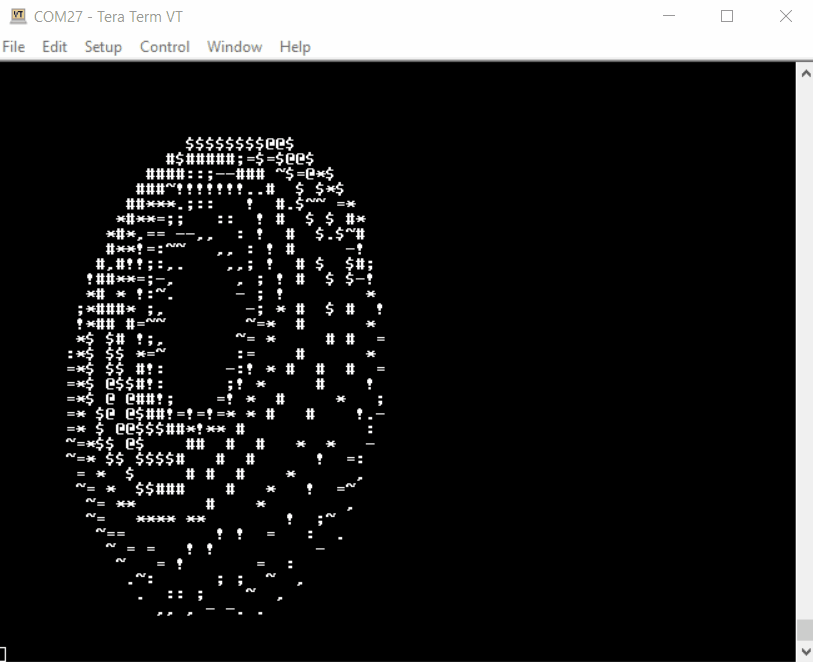Donut yes… but so slow

# First optimization — Sine lookups

If we take a look at the code, there are 2 heavy math functions (sin and cosine). A old trick in embedded systems is to pre-calculate a lookup table of sine’s and store it instead of calculating it on the fly.

If you look carefully at the math, the for() loop scales upto 2*PI(~6.28) in minimum steps of 0.02. All that means is that I can have a neat little lookup table of floats with 314 members and where one step equals to 0.02 i.e.,

If I wanted sin(0.02); I would do a sine_lookup which has the precalculated floating point lookup value.

Making a simple change to the sine and cosine functions drastically improves the rendering time by about 2x;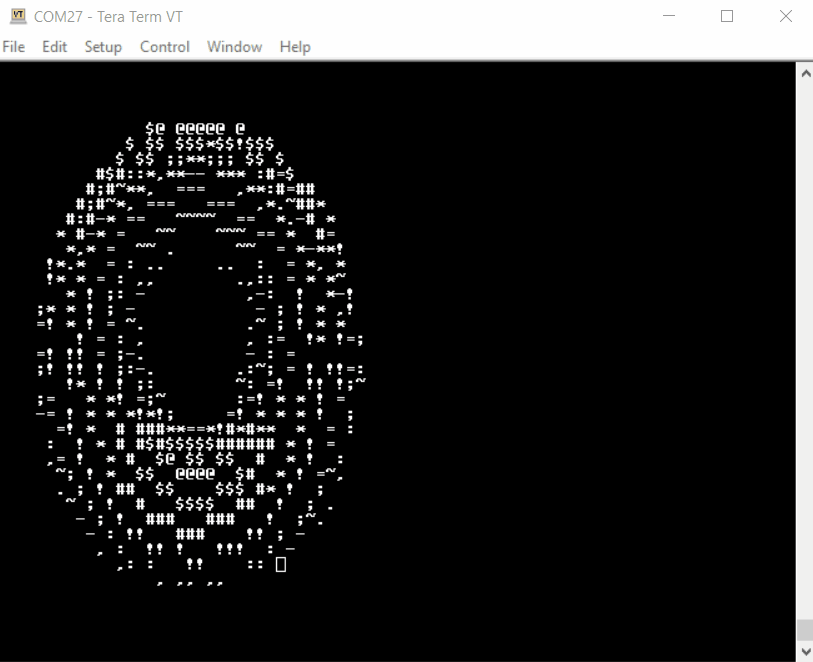Better, but can we do more?

# Pure Fixed point math — Q Notation change

Since the current Shakthi RISCV doesn’t have a dedicated floating point acceleration engine, the compiler implements a soft float. Float Multiplies, divides and adds are all very computationally expensive; but very well optimized if we can make it fixed point math.

Another DSP/embedded systems trick is to convert floating points to Q notation.

So, we can shift the floating point number by a few bits(basically just cheap multiplications) and perform math operations on them with some caveats. All we need to do is make sure we shift the values back before we use them.

The Q-notation is pretty awesome once you know how to use it but it’s beyond the scope of this little article; maybe some other time

I went ahead and implemented a couple of basic q based multiplier and divisions and refactored code. This gives a significant bump in render speeds and it’s finally beginning looking a lot smoother.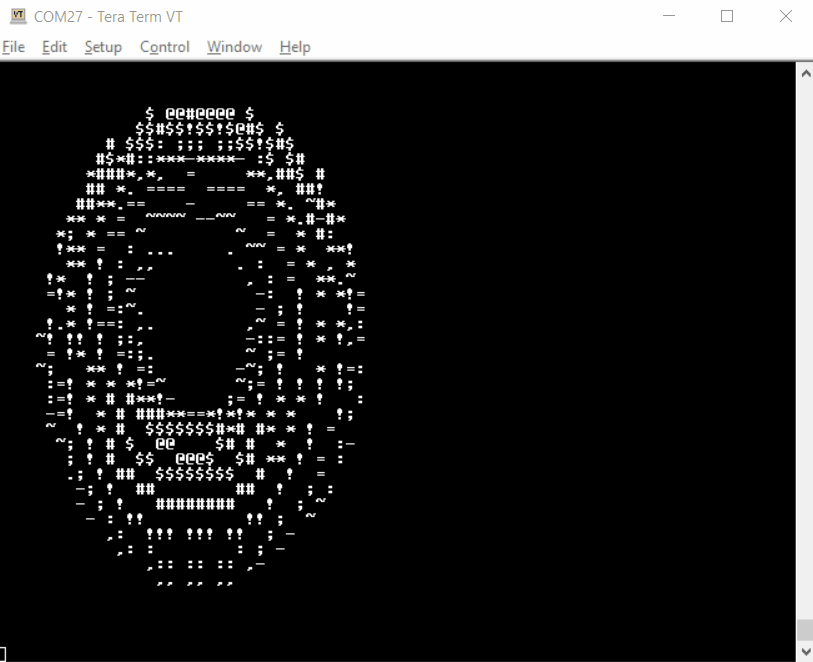Donut using Q15.16 math….woohoo!

# Just a little more — moving away from 64-bit math and improving the print statements

As a last challenge, I wanted to see if I could really push it to the edge with a couple of more ideas I had.

The first idea is that the original Q15.16 multiplication and division requires you to use 64-bit variables for storing the result before you can truncate the lower 32-bits. I suspect I wouldn’t need to do this if I coded in direct RISCV assembly(looks like MUL truncates automatically) but I didn’t go down that path.

Instead, I decided to simply reduce the amount of precision I have by making it a Q7.8 number making it a ‘int16’ instead of a ‘int32’.

Note: I had to trial and error my way to find the ideal fit as there’s a very strong trade-off between the precision and the upper limit of the value. If you do plan to use low bit numbers like this; it’s preferred that you normalize your values instead of being lazy like me :)

The second idea is to improve the printing logic, the original is shown below

`for (int j = 0; j < SCREEN_HEIGHT; j++) {    for (int i = 0; i < SCREEN_WIDTH; i++) {      putchar(output[i][j]);    }    putchar('\n');  }`

For people familiar with embedded systems; the putchar() function is generally much slower than the printf() function. Instead, if we can bake-in new-line characters(\n) at the end of each row and correctly terminate the 2-D string array with a NULL character, you can get away with just a single printf() function.

After these two little optimizations we get something which is pretty awesome looking if I do say so myself.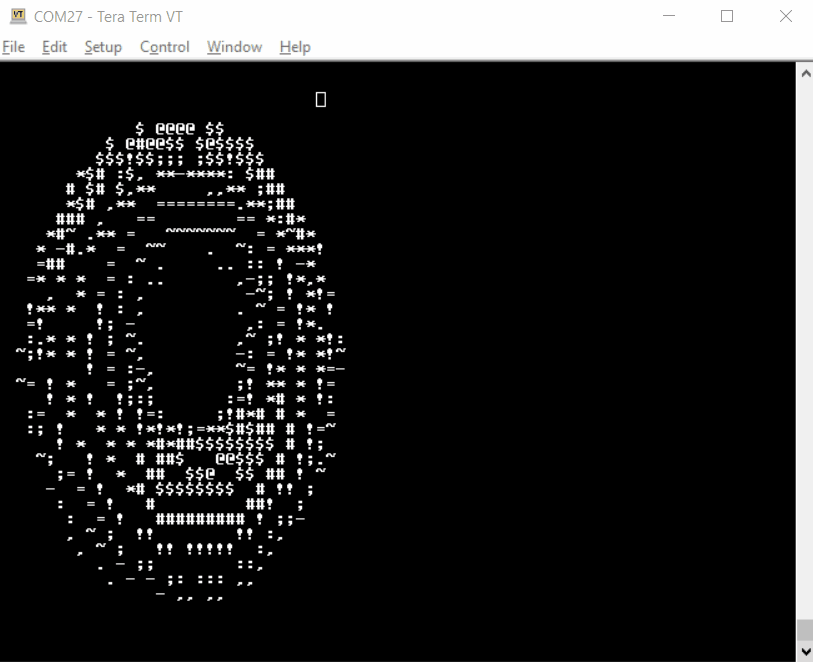Q7.8 and improved printing; now I’m really done

# Closing Thoughts

While I spent entirely too much time on donuts; it was a great deal of fun recalling some old tricks taught by some older engineering colleagues.

In general, I think the Shakti platform holds a lot of promise once it goes over it’s initial growing pains. I still haven’t had a chance to play with PWM’s and other serial communication interfaces but that’s for another time. I’m also definitely interested in trying out Zephyr on this platform so I may have one more part in this series.

## The Startup

Get smarter at building your thing. Join The Startup’s +787K followers.

## Sign up for Top 10 Stories

### By The Startup

Get smarter at building your thing. Subscribe to receive The Startup's top 10 most read stories — delivered straight into your inbox, once a week. Take a look.

By signing up, you will create a Medium account if you don’t already have one. Review our Privacy Policy for more information about our privacy practices.

Check your inbox
Medium sent you an email at to complete your subscription.

## The Startup

Get smarter at building your thing. Follow to join The Startup’s +8 million monthly readers & +787K followers.

Written by

## Sree Harsha Angara

Embedded system tinkerer and IoT Security Applications expert## The Startup

Get smarter at building your thing. Follow to join The Startup’s +8 million monthly readers & +787K followers.